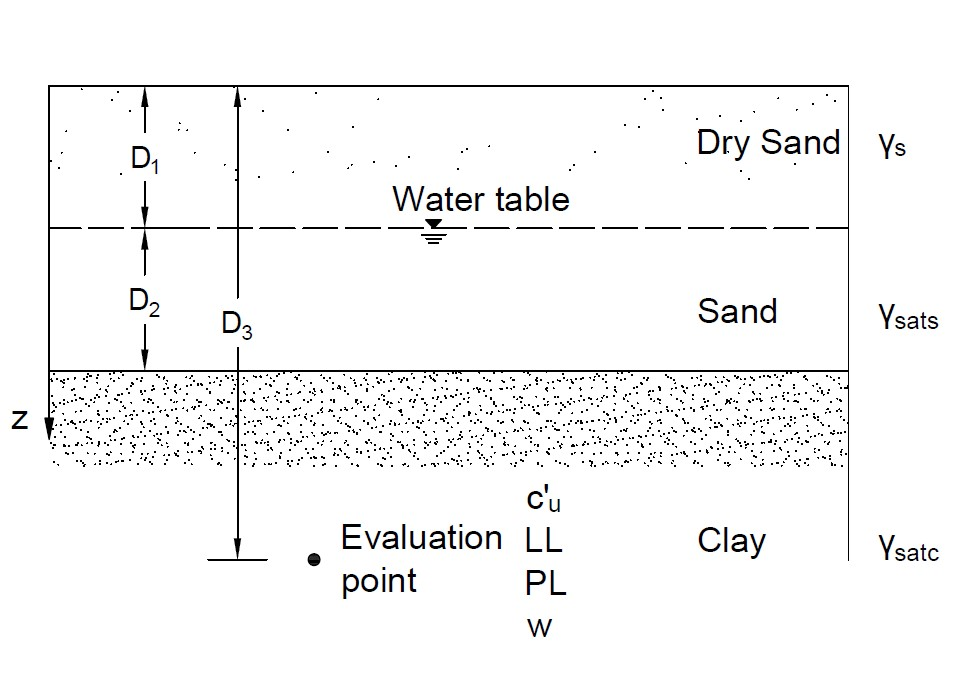# Vane Shear Test Tool## Geometry of Vane Shear Test## Tips

• This tool is for obtaining mechanical properties of clays through the vane shear test (VST), as shown in the figure above.
• All the input parameters should be positive.
• The unit weight of water is 9.8 kN/m3, and the atmospheric pressure is 100 kPa.
• The evaluation point should be in the clay layer (D3>D1+D2).
• Pay attention to the dimensions of the measured torque and the height of vane.
• The diamater of the vane D = 0.5H. Water content w is only used in Hansbo Method for β.
• The Liquid limit LL should be larger than the Plastic limit PL.
• The estimations of coefficients λ and β proposed by different researchers are used for calculating preconsolidation pressure σ′c and overconsolidation ratio OCR.
• For "nan", "0" or "inf" displayed in Results, please check your input parameters.

D1 (m)
D2 (m)
D3 (m)
γ_s (kN/m3)
γsat_s (kN/m3)
γsat_c (kN/m3)
T (kN.m)
H (cm)
LL (%)
PL (%)
w (%)

## Output Results

• Morris & Williams Method
Corrected undrained cohesion
cu (kPa) =
Preconsolidation pressure
σ′c (kPa) =
Use β by Mayne&Mitchell Method
OCR =
Use β by Hansbo Method
OCR =